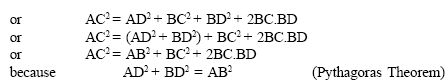Email us to get an instant 20% discount on highly effective K-12 Math & English kwizNET Programs!

#### Online Quiz (WorksheetABCD)

Questions Per Quiz = 2 4 6 8 10

### High School Mathematics - 28.14 Pythagorean Theorem - Examples

 Theorem: In a right angled triangle, the square on the hypotenuse is equal to the sum of the squares of the other two sides. If the length of the hypotnuse is c and the lengths of other two sides are b and a then according to the pythogorean theorem: c2 = a2 + b2Converse: In a triangle if the square of one side is equal to the squares on the sums of the other two sides, then the angle opposite to the first side is a right angle. To prove: AB2 + BC2 = AC2Example: Prove that the sum of the squares of the sides of a rhombus is equal to the sum of the squares of the diagonals.Directions: Solve the following problems. Also write at least 10 examples of your own.
 Q 1: The sides of a triangle are 7cm, 24cm, 25cm, check if this is a right angled triangle.noyes Q 2: Triangle PQR is equilateral. QR = 30 units, B is the midpoint of QA. QA is perpendicular to PR. What is the length of PB? 24 cm19.84 cm23 cm Q 3: The sides of a triangle are 50cm, 80cm, 100cm, check if this is a right angled triangle.yesno Q 4: A ladder 20m long reaches height of 16m above the ground. Find the distance of the foot of the ladder from the wall.13 m14 m12 m Q 5: Which of these is a Pythagorean triplet?15, 8, 513, 12, 58, 9, 13 Q 6: Two poles 6m and 11m stand on a plane ground. If the distance between their feet is 12m, determine the distance between their tops.13 m11 m12 m Q 7: A person goes 10 m due east and then 30m due north. Find his distance from the starting point.15m12m10m Q 8: The sides of a triangle are 3cm, 8cm, 6cm, check if this is a right angled triangle.noyes Question 9: This question is available to subscribers only! Question 10: This question is available to subscribers only!